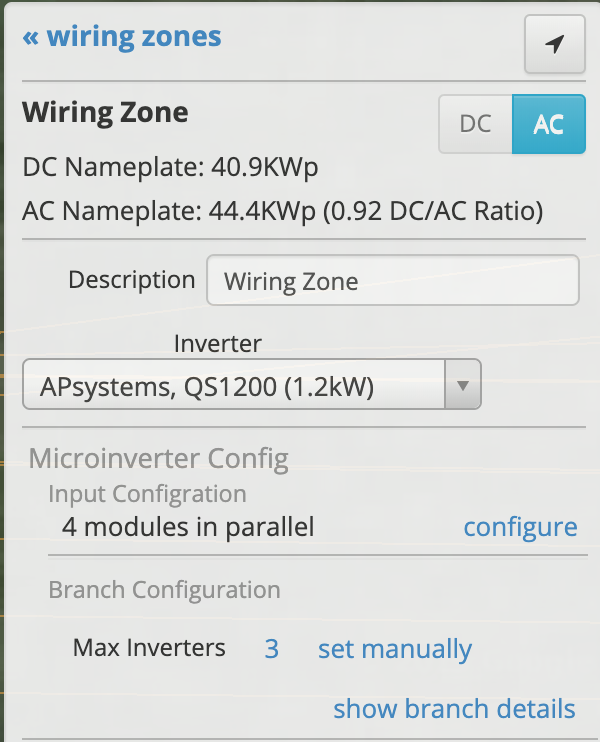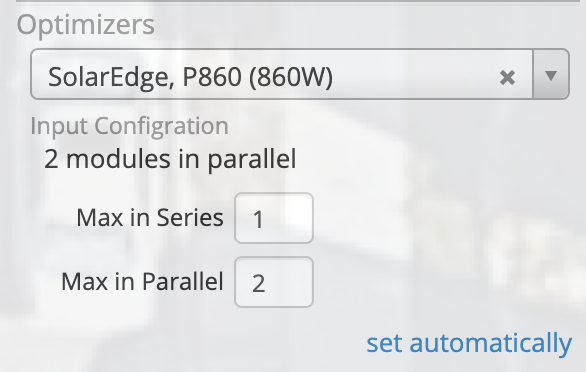# Multiple-Input Micro Inverter and Optimizers

Throughout this article we will use Module-Level Power Electronics (MLPE) to describe micro inverters and optimizers as a category.HelioScope will automatically calculate the number of modules that connect into a MLPE, but you can override these calculations to manually set the number of inputs into the device. This article discusses the different configuration options for MLPE's.

In order to calculate the electrical interactions correctly, HelioScope needs to know whether the modules connected into the MLPE are connected in parallel or series. By default, HelioScope will maximize the number of modules into a device and find the limits based on the voltage, current, and power limits of the device .

### Micro Inverter Example:

In the screenshot above, the user has selected APsystems QS1200 micro inverters with the following specifications:

• Max. Power: 1.2kW
• Max. Voltage: 60V
• Max. Current: 48A (12A per input)

To determine the maximum number of modules HelioScope will look at the max power of the micro inverter (1200W) and multiply by a 1.25 DC/AC ratio (1500W). The module in this example is 280W so the max power would support up to 5 modules.

Next, we look at the max voltage to determine how many modules can be connected in series. The Voc of the module is 39.2V so our maximum series length into the MLPE is 1.

Last, we look at current to determine how many series circuits can be connected in parallel into the MLPE. In this case the module Isc is 9.4A so the maximum parallel connections is 1 per input, or 4 total.

If you have a max branch circuit of 3 micro inverters your maximum modules per branch will be 12 modules.

### How Are Odd Module Counts Handled?

The input configuration describes the maximum number of modules that can be connected to an MLPE, and HelioScope will use partially-loaded MLPE's to connect any spare modules in a branch.

If we have 11 modules in our design and a configuration like the example above, HelioScope will set up the branch with 2 micro inverters with 4 modules each, and 1 micro inverter with 3 modules.

### Overriding Automatic CalculationsHelioScope's calculation will get you a reasonable answer very quickly, but you may want to manually override the automatic calculations for a given application. In that case, click the Configure button to the right of the Input Configuration description.

You will now be able to set the number of modules into the device and whether the modules are connected in parallel or in series. HelioScope will provide a warning if you exceed any of the input maximums, but it will still allow you to model the system.

Note: setting Max. in Series to 2 and Max. In Parallel to 2 will result in 2 strings of 2 (total of 4 modules) connected into the device.